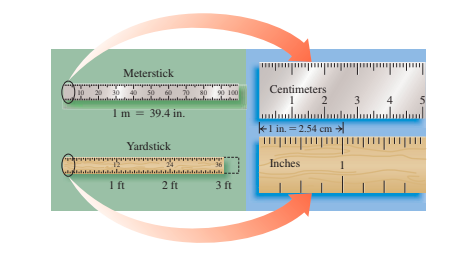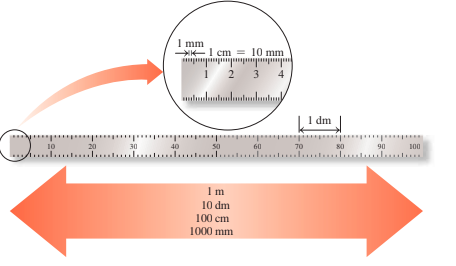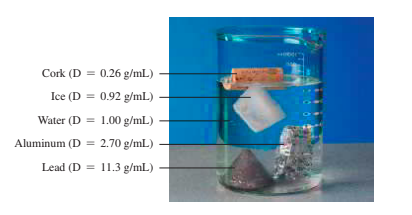# 2.1 - Units of Measurement

• Physical amounts in science are described in units of the metric or international units system (SI).

• Some of the key units include volume liter (L), length meter (m), gram (g), weight kilogram (kg), temperature degree Celsius (°C) and Kelvin (K), and time second units.# 2.2 - Measured Numbers and Significant Figures

• Any number obtained by using a measuring instrument is a measured number.

• An accurate number is obtained by counting items or from a definition.

• The numbers reported in measurement including the estimated number are significant figures.

• Zeros are not significant before the decimal number or at the end of the non-decimal number.

# 2.3 - Significant Figures in Calculations

• The final answer is written in multiplication and division to have the same number of significant numbers as the most insignificant measurements.

• The final answer has been written so that there is the same number of decimal places as the least decimal places measurement.

# 2.4 - Prefixes and Equalities

• A prefix located before a metric or SI device changes the unit's size by 10.

• Prefixes like centi, milli, and micro are smaller, prefixes like kilograms, megagrams, and there are large.

• The relationship between two units, measuring identical volume, length, weight, or time, shows equal equality.# 2.5 - Writing Conversion Factors

• To express a relation in a fraction, conversion factors are used for any relationship in the metric or in the US system, two conversion factors may be written.

• By speaking matching components in 100 parts, a percentage is written as a conversion factor.

# 2.6 - Problem Solving Using Unit Conversion

• Conversion factors are helpful if a quantity in one unit is changed to a quantity in another one.

• A given unit is multiplied by one or more transformation factors in the problem-solving process which cancels the units until the answer has been obtained.# 2.7 - Density

• The volume is usually g/mL or g/cm3, a ratio of its mass to the volume of the substance.

• Conversion factors between mass and volume of a material can be written using the units of density

• The density of a substance is comparable to the water density, 1.00 g/mL.